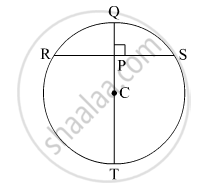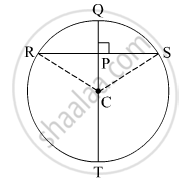Advertisement Remove all ads

# In the Given Figure, C is the Centre of the Circle. Seg Qt is a Diameter Ct = 13, Cp = 5, Find the Length of Chord Rs. - Geometry

Diagram

In the given figure, C is the centre of the circle. seg QT is a diameter CT = 13, CP = 5, find the length of chord RS.Advertisement Remove all ads

#### SolutionJoin RC and CS.
Perpendicular drawn from the centre of the circle to the chord bisects the chord.
So, RP = PS
In Δ RPC,

RP2 + PC2 = RC2

⇒ RP2 + 52 = 132

⇒ RP2 = 169 - 25 = 144

⇒ RP = 12 cm

Thus, RP = PS = 12 cm
So, RS = RP + PS = 12 + 12 = 24 cm.

Is there an error in this question or solution?
Advertisement Remove all ads

#### APPEARS IN

Advertisement Remove all ads
Advertisement Remove all ads
Share
Notifications

View all notifications

Forgot password?